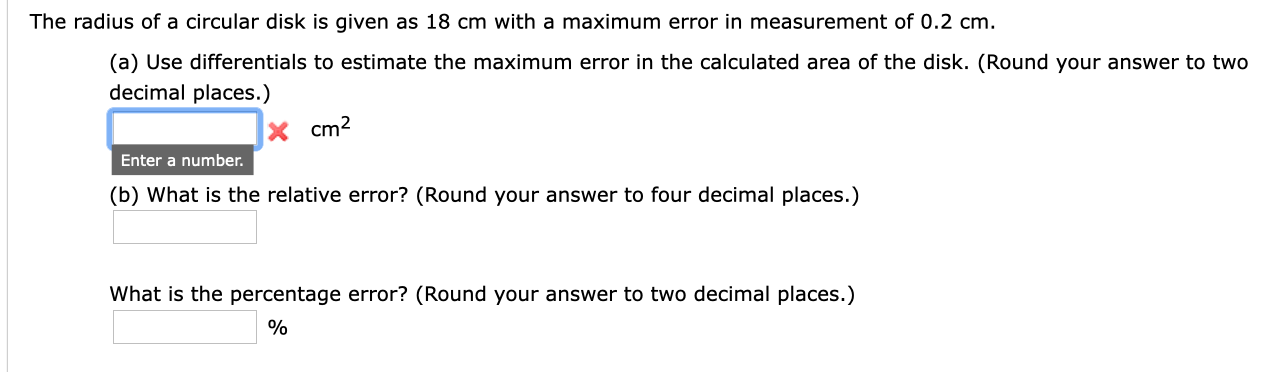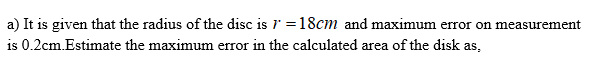# The radius of a circular disk is given as 18 cm with a maximum error in measurement of 0.2 cm(a) Use differentials to estimate the maximum error in the calculated area of the disk. (Round your answer to twodecimal places.)cm2Enter a number.(b) What is the relative error? (Round your answer to four decimal places.)What is the percentage error? (Round your answer to two decimal places.)

Question
183 viewshelp_outlineImage TranscriptioncloseThe radius of a circular disk is given as 18 cm with a maximum error in measurement of 0.2 cm (a) Use differentials to estimate the maximum error in the calculated area of the disk. (Round your answer to two decimal places.) cm2 Enter a number. (b) What is the relative error? (Round your answer to four decimal places.) What is the percentage error? (Round your answer to two decimal places.) fullscreen
check_circle

Step 1Step 2

b) Obtain the relati...

### Want to see the full answer?

See Solution

#### Want to see this answer and more?

Solutions are written by subject experts who are available 24/7. Questions are typically answered within 1 hour.*

See Solution
*Response times may vary by subject and question.
Tagged in

### Other